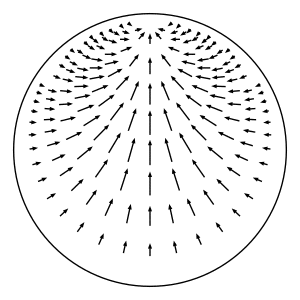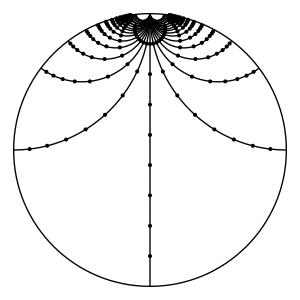# Horocyclic flow

In hyperbolic geometry, the horocyclic flow on a hyperbolic surface is a canonical flow on the unit tangent bundle of the surface that moves tangent vectors sideways along the horocycle whose center is in the direction of the tangent vector.This figure illustrates the horocyclic flow in the Poincaré disk model of the hyperbolic plane. The figure shows the flow restricted to the subspace of unit tangent vectors that point to the boundary point at the top (i.e. the complex point $$i$$ in the disk model). This subspace is a section of the unit tangent bundle of the plane and is preserved by the flow.This figure shows how the horocyclic flow acts over time on the unit tangent vectors that lie along a geodesic. In particular, you can see that the flow doesn't preserve these geodesics. Rather, the tangent vectors along a geodesic are taken to points along a curve equidistant from the initial geodesic.

## Definition

More precisely, let $$S$$ be a complete oriented hyperbolic surface, and let $$T^1(S)$$ denote the unit tangent bundle of $$S$$. Consider a point $$p = (x, v)\in T^1(S)$$, where $$x\in S$$ and $$v$$ is a unit tangent vector based at $$x$$. We define the horocyclic flow by describing where the flow takes $$p$$ at time $$t\in \mathbb{R}$$. We use $$h$$ to denote the horocyclic flow on $$S$$, and we let $$h_t: T^1(S)\to T^1(S)$$ denote the mapping at time $$t$$.This figure illustrates the definition of the horocyclic flow in the universal cover of a hyperbolic surface, using the Poincaré disk model of the hyperbolic plane.

As shown in the figure above, lift $$p$$ to a point $$\widetilde{p} = (\widetilde{x}, \widetilde{v})$$ in the unit tangent bundle of the hyperbolic plane $$\mathbb{H}$$. (The hyperbolic plane is the universal cover of $$S$$.) Let $$c$$ denote the point in the boundary at infinity of $$\mathbb{H}$$ that $$\widetilde{p}$$ points to. In other words, the point $$c$$ is the endpoint of the geodesic ray that starts at $$\widetilde{x}$$ and whose tangent vector is $$\widetilde{v}$$. Consider the oriented horocycle $$C$$ in $$\mathbb{H}$$ with center $$c$$ and that passes through $$\widetilde{x}$$ from left to right when facing in the direction of $$\widetilde{v}$$. (This is where we use the orientation of $$S$$.)

Define $$\widetilde{p}_t$$ to be the element $$(\widetilde{x}_t, \widetilde{v}_t)\in T^1(\mathbb{H})$$, where $$\widetilde{x}_t$$ is the result of moving $$\widetilde{x}$$ along $$C$$ for time $$t$$ at unit speed (measuring distance relative to the metric that $$C$$ inherits from $$\mathbb{H}$$), and $$\widetilde{v}_t$$ is the unit tangent vector based at $$\widetilde{x}_t$$ pointing towards the same boundary point $$c$$. Note that the distance between $$\widetilde{x}_t$$ and $$\widetilde{x}$$ as measured in $$\mathbb{H}$$ will be strictly less than $$t$$ because $$C$$ is not a geodesic. (This distance in $$\mathbb{H}$$ is $$2\sinh^{-1}(t / 2) < t$$.) Project $$\widetilde{p}_t$$ down to $$p_t\in T^1(S)$$ to get $$h_t(p)$$. This defines a well-defined flow because the construction doesn't depend on the choice of lift nor on the time $$t$$.